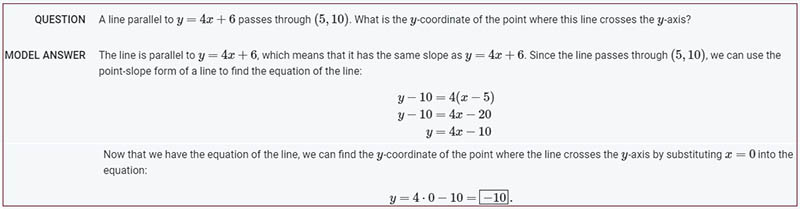# AI for Solving Quantitative Reasoning Problems – Minerva

Google AI Introduces Minerva: A Natural Language Processing (NLP) Model for solving  Mathematical Questions

Solving mathematical and scientific questions was one of the area where AI cannot prove by itself so far. This is mainly because for solving Quantitative , it needs combination of skills and there is not a straigt rule for parsing the question correctly. Main challenges for on parsing mathematical notation and generate step-by-step solutions. Now Google introduced Minerva, a language model capable of solving mathematical and scientific questions using step-by-step reasoning. As per the google, its not using the calculator directly, instead the model parses and answers mathematical questions using a mix of natural language and mathematical notation. Google launched Minerva training on a 118GB dataset of scientific papers from the arXiv preprint server and web pages having mathematical expressions which can be read by LaTex formats. Minerva’s technique include chain of thought or scratchpad prompting. Minerva gives its different possible outputs, multiple solutions are generated by sampling.

Sample resultAuthor: user